﻿ 从SIL2系统设计和SIL3系统设计的区别了解SIL系统设计

# 从SIL2系统设计和SIL3系统设计的区别学习SIL系统设计

2019/12/2 0:46:31 人评论 次浏览 分类：DCS  文章地址：http://yunrun.com.cn/tech/2821.html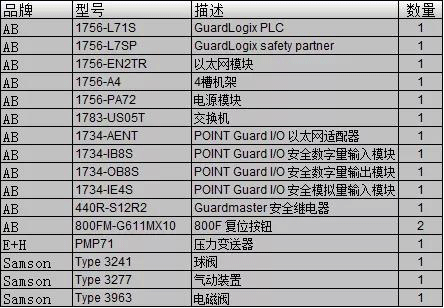SIL2等级系统硬件接线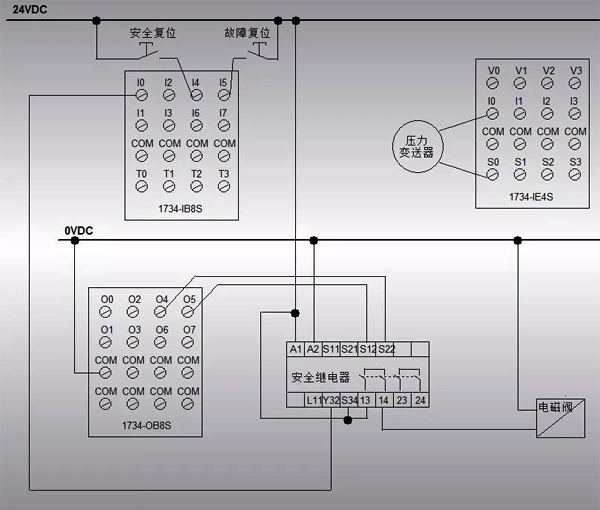SIL2等级系统软件配置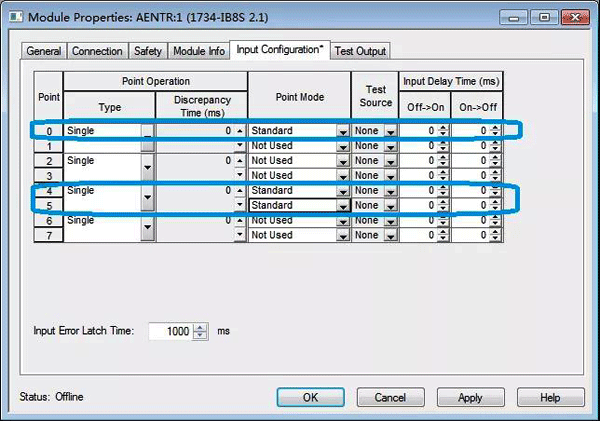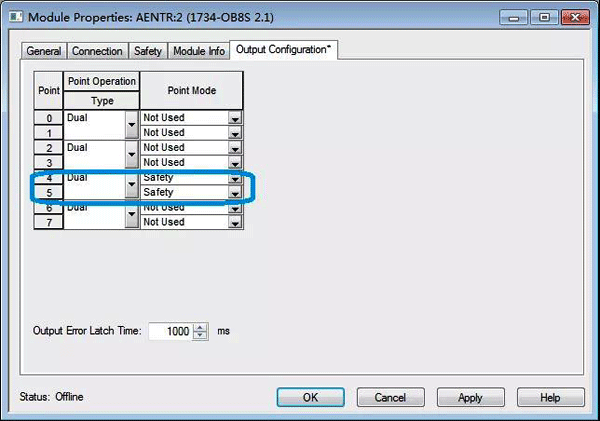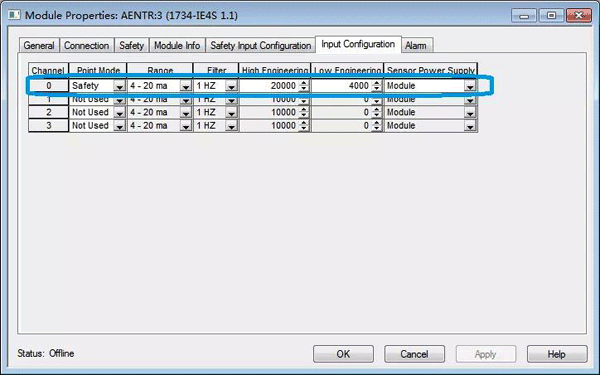SIL等级计算

PFD(系统)=PFD(输入)+PFD(逻辑)+PFD(输出)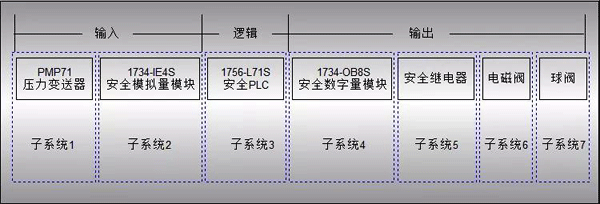PFD(系统)=PFD(子系统1)+PFD(子系统2)+PFD(子系统3)+PFD(子系统4)+PFD(子系统5)+PFD(子系统6)+PFD(子系统7)
=2.86E-04 + 2.40E-06 + 3.50E-05 + 2.13E-05 + 5.68E-04 + 7.79E-06 + 2.40E-04
=1.16E-03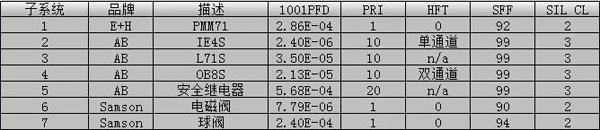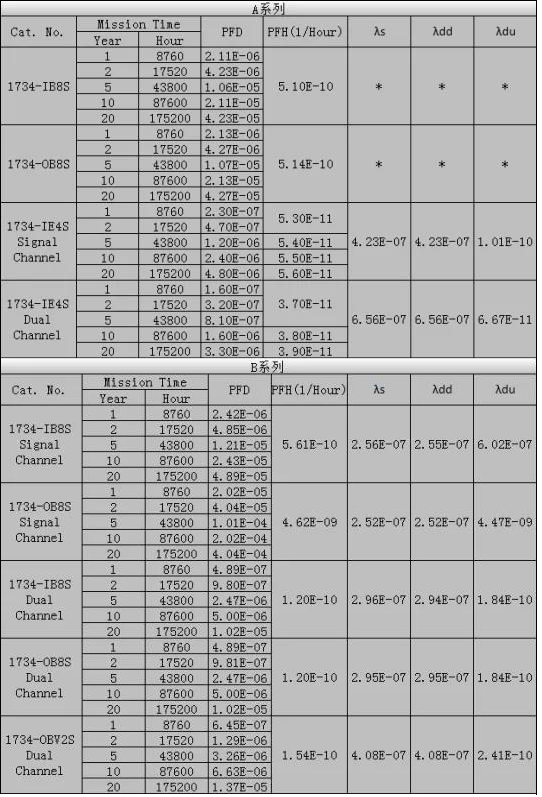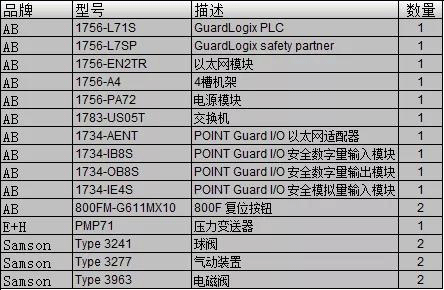SIL3等级系统硬件接线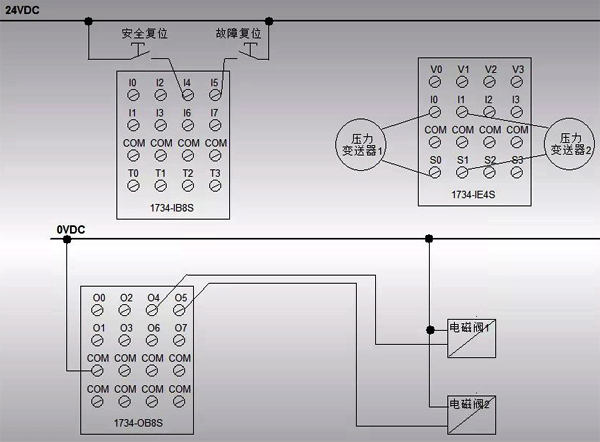SIL3等级系统软件配置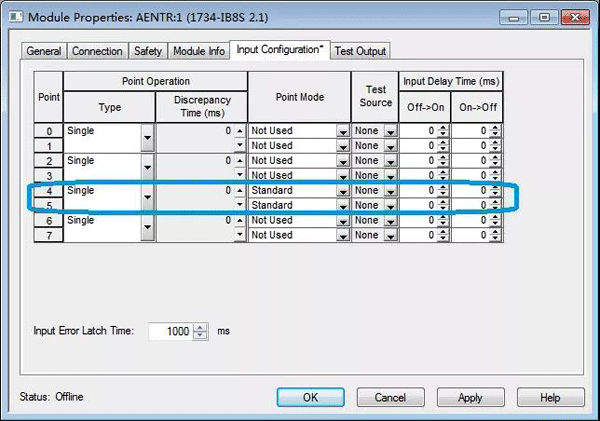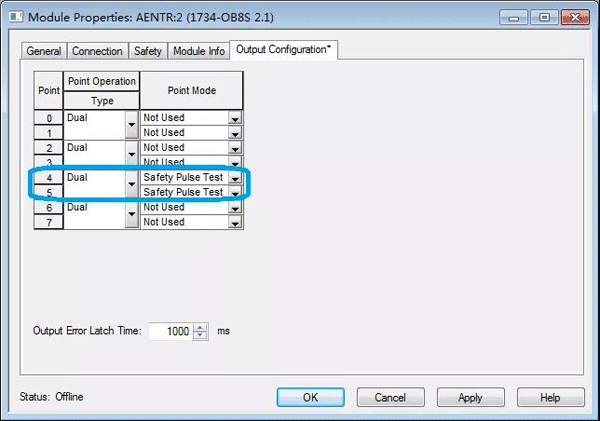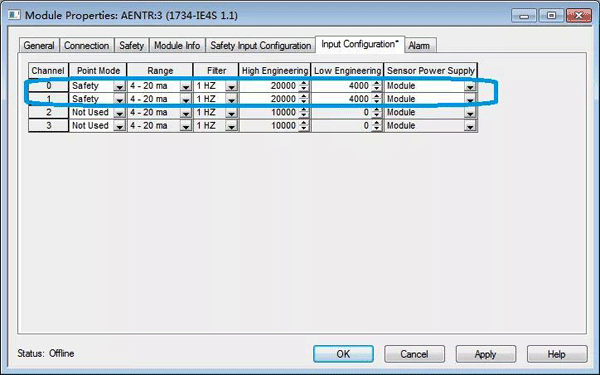SIL等级计算

PFD(系统)=PFD(输入)+PFD(逻辑)+PFD(输出)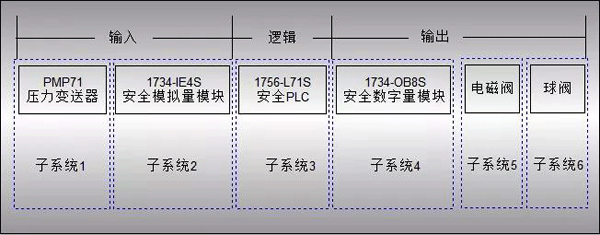分别在E+H，AB，Samson官网查询产品手册和安全数据，汇总到如下表格。所以我们计算出：
PFD(系统)=PFD(子系统1)+PFD(子系统2)+PFD(子系统3)+PFD(子系统4)+PFD(子系统5)+PFD(子系统6)
=2.86E-05 + 2.40E-06 + 3.50E-05 + 2.13E-07 + 7.79E-07 + 2.40E-05
=1.12E-04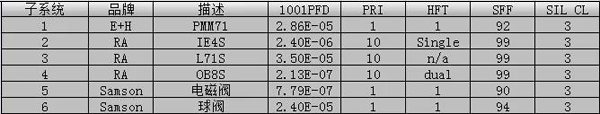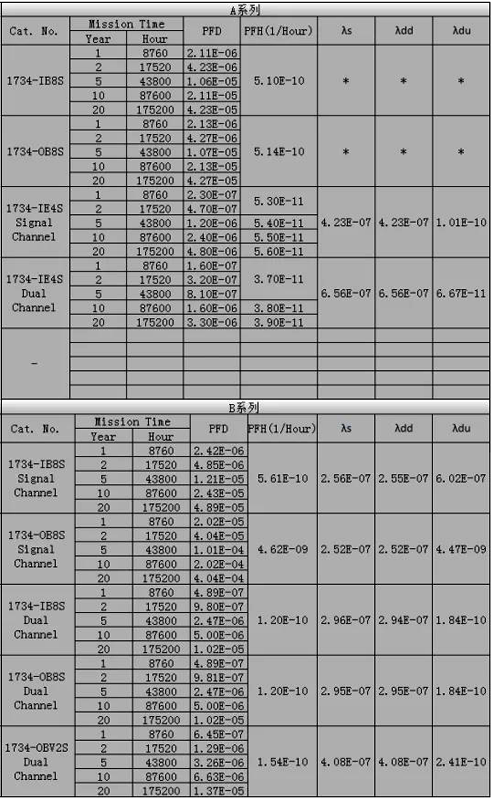SIL定级与验证知识十问十答
SIL等级是怎么确定的?依据是什么？
 与在线工程师QQ交流 到论坛与网友交流 向昌晖仪表网投稿 免费获得2000元电子书

客户姓名：